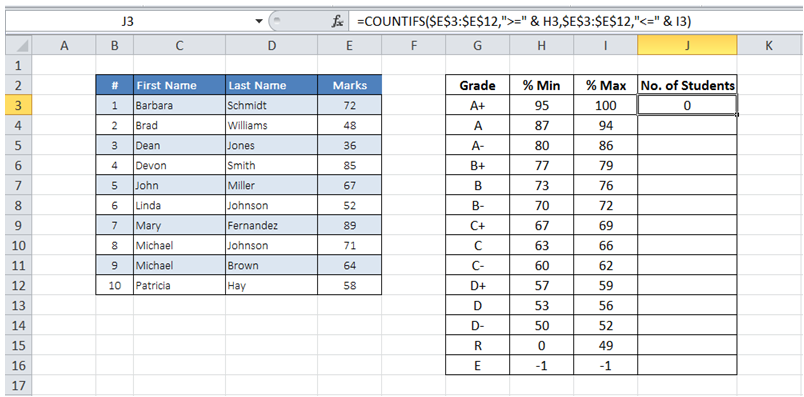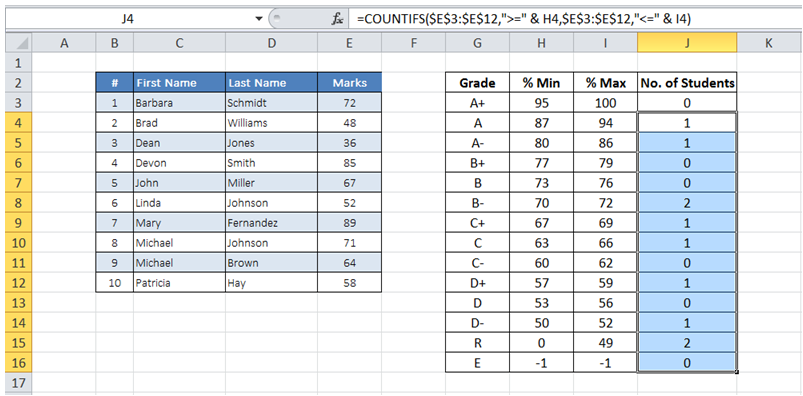## Count multiple condition in range in Excel

For example, I want to get the count of number of students that fall in each grade given their marks and grading table.

To do it in Excel, here is the answer:

a) Enter the formula =COUNTIFS(\$E\$3:\$E\$12,">=" & H3,\$E\$3:\$E\$12,"<=" & I3) where E3:E12 has marks data, H3 and I3 represent the minimum and maximum marks for a given grade.

The above formula checks and counts the number of marks that fall with value contained in H3 and I3 (both numbers inclusive).b) Copy the formula as needed for the remaining grade definitions.